# AP Board 4th Class Maths Solutions 3rd Lesson Addition

## AP State Syllabus 4th Class Maths Solutions Chapter 3 Addition

Play and Win :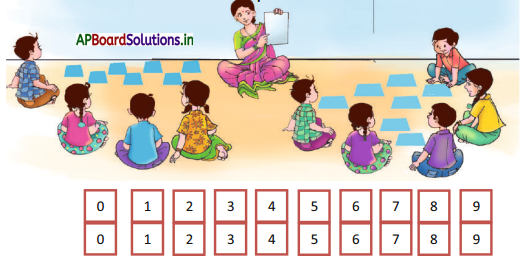• Divide the students into 2 groups.
• Supply each group 2 sets of number cards from 0 to 9.
• Each student will take one set.
• Flip the cards to hide the numbers.
• Jumble the cards to change the order.
• One student from the first group selects 4 cards from second set of numbers and makes another four digit number.
• Then they add two numbers and write down the sum in the note book.
• Every group does the same. .
• The group which gets the largest sum will get 1 point.
• After 10 rounds who got more points they will be declared as winners.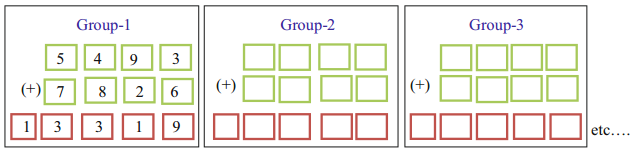This is an Addition machine. It has a fixed 4-digit number 4,728. If you place any 4 digit number in the machine, it is added to 4728 and the sum if displayed on the screen. You should place a 4-digit number of your choice and find the number displayed by the machine.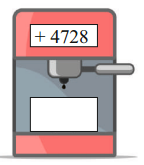Question 1.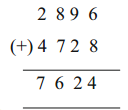Question 2.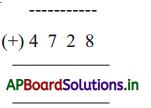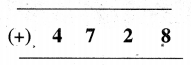Question 3.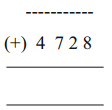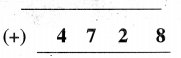Question 4.Question 5.Textbook Page No. 34

Do this

a)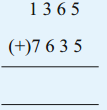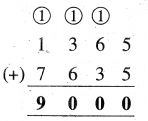b)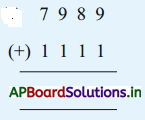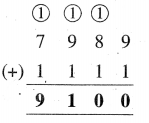c)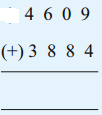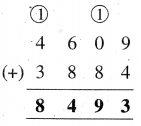d)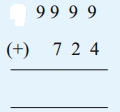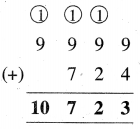Question 2.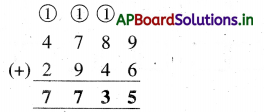Question 3.
Find 7645 + 5895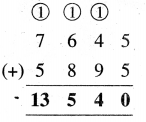Example – 2:

In another shop there are two types of bicycles namely gear bicycles and small bicycles.

Now you find out the two bicycles whose cost would be less than 10,000 and fill the table. The cost of each bicycle is given inboxes.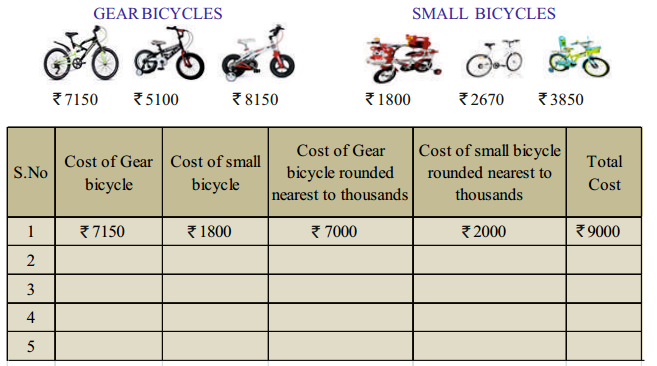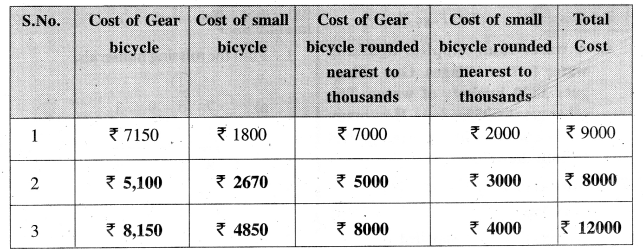Textbook Page No. 34

Do this

Question 1.
Estimate the sum in each case and tick (✓) on the correct box which is nearest to its sum.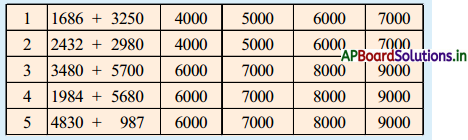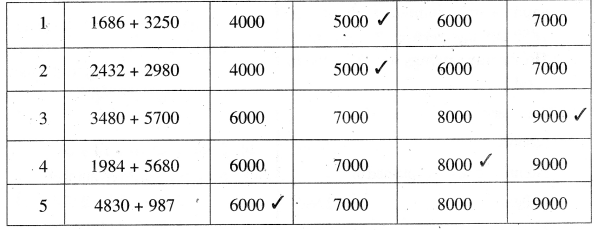Question 2.
Estimate the sum.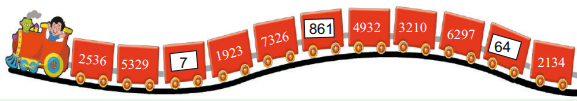There is a kids train. All the compartments have their numbers on them. Find the pairs of compartments whose sum is greater than 8000.
(2536, 7326), (5329, 7326), (7326, 861), (2536, 6297), (5329, 4932), (5329, 3210), (5329, 6297), (1923, 7326), (1923, 6297), (7326, 4932), (7326, 3210), (7326, 6297), (7326, 2134) (4932, 3210), (4932, 6297), (3210, 6297), (6297, 2134),

Textbook Page No. 37

Do this

Question 1.
A water tank supplies drinking water to two villages. One village gets 3870 buckets of water. The other gets 5,295 buckets. How many buckets of water in total are supplied to both the villages ?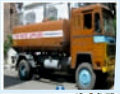Solution:
Number of buckets supplied to first village = 3,870
Number of buckets supplied to second village = 5,295
Number of buckets supplied to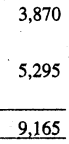Totally = 9,165Question 2.
On an independent day, 7,305 saplings wree planted in schools and 2859 saplings were planted in offices. How many saplings were planted in total ?
Solution:
Number of saplings planted in schools = 7,365
Number of saplings planted in Offices = 2,895
Number of saplings planted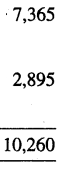Totally = 10,260

Textbook Page No. 38, 40

Try this

Make word problems for the following:

a) 6,854 + 3,521
Solution:
Latha had 6,854 bangles and Prasanna had 3,521 bangles. How many bangles were there in all ?

b) 5,340 + 3,564
Solution:
A fruit seller sold 5,340 apples and 3,564 oranges in a day.How many fruits did he sell?c) 4,563 + 8,520
Solution:
Sirisha has 4,563 pencils and Sohan has 8,520 pencils. How many pencils are there altogether ?

1. Find the missing numerals.

a)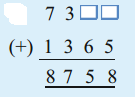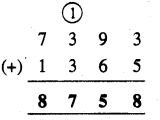b)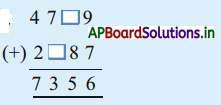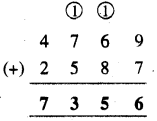2. Find the sum by suitable re-grouping.

a) 740 + 320 + 260 + 2,680
Solution:
We have to re-group the given in the following way.
= 740 + 260+ 320 + 2,680
= 1000 + 3,000
= 4,000b) 5,986 + 2,976 + 14 + 24
Solution:
Re-group the given numbers.
= 5,986+ 14 + 2,976 + 24
= 6,000 + 3,000
= 9,000

c) 4,893 + 894 + 106 + 107
Solution:
Re-group the given numbers.
= 4,893 + 107 + 894 + 106
= 5,000 + 1,000
= 6,000

Exercise – 3.1

a)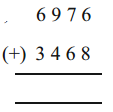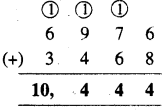b)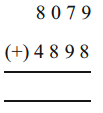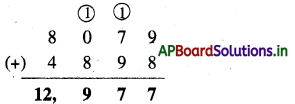c)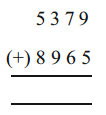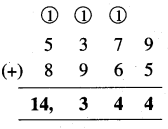d)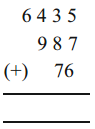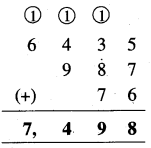a)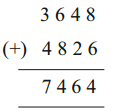Givenis wrong
Justification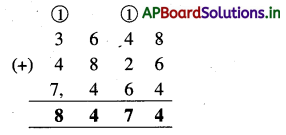b)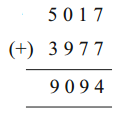Givenis wrong
Justification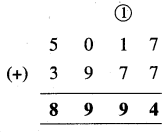c)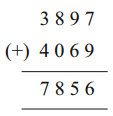Givenis wrong
Justification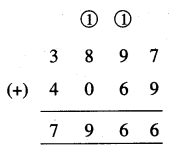3. Write word problems for the following additions:

a) 3,268 + 5,634 = ?
Solution:
The balloon seller had 3,268 blue balloons and 5,634 red balloons. How many balloons did he have in all ?b) 6,240 + 5,425 = ?
Solution:
A gift box has 6,240 chocolates and 5,425 lollipops. How many things are there in the box ?

4. Fill in the blanks.

a) 632 + 984 = 984 + _________
Solution:
632

b) 2,735 + __________ = 2,569 + 2,735
Solution:
2.569

Question 5.
A number exceeds 6897 by 5,478. What is that number?
Solution:
Sum of 6897 + 5478
∴ That number = 12,375

Question 6.
Veerayya sold maize for ₹ 5,397 and pearl millets for ₹ 3,849 in a village fair. How much amount did he get ?
Solution:
Selling price of maize = ₹ 5,397
Selling price of millets = ₹ 3,849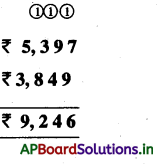Total amount he got = ₹ 9,246

Question 7.
Madhav produced 3,985 watermelons in his field. Vijender produced 854 more than Madhava’s. What is the total number of watermelons produced by him?
Solution:
Watermelons produced by Madhav = 3,895
Vijendar produced 854 more than Madhav.
∴ Watermelons produced by Vijendar
= 3,985 + 854
= 4,839.Question 8.
The number of visistors to Arasavalli temple on three consecutive days in Karthika masam is 3842, 2642 and 1,958 respectively. What is the total number of visitors during these days ?
Solution:
Number of visitors visited on First day = 3,842
Number of visitors visited on Second day = 2,642
Number of visitors visited on Third day = 1,958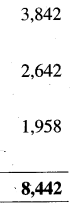Total number of visitors during these days = 8,442

Multiple Choice Questions

Question 1.
Nearest to thousand to the number 7,250 is
A) 7,000
B) 7,500
C) 8,000
D) 7,750
A) 7,000

Question 2.
The sum of 1,984 + 2,020 to nearest thousand number
A) 2,000
B) 3,000
C) 4,000
D) 5,000
C) 4,000Question 3.
3,265 + 2,678 = ……………… + 3,265
A) 2,678
B) 3,265
C) 5,943
D) None
A) 2,678

Question 4.
There are 2,475 girls and 3,950 boys in a school. How many students are there in all ?
A) 6,425
B) 6,000
C) 6,500
D) 7,000